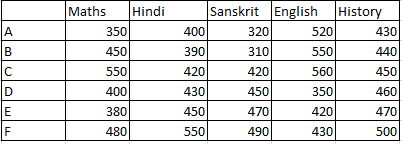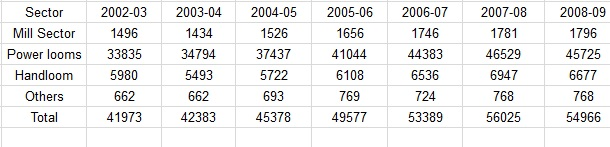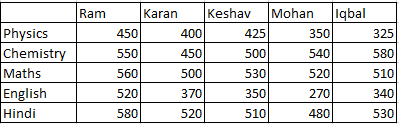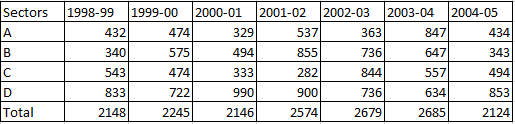# Data Interpretation Questions for RRB Group-D

0
448

## Data Interpretation Questions for RRB Group-D

Download Top 15 RRB Group-D  Data Interpretation  Questions and Answers PDF. RRB Group-D Data Interpretation questions based on asked questions in previous exam papers very important for the Railway Group-D exam.

Instructions

Given table shows marks of 6 students in 5 subjects and marks have been given in out of 600 marks. Study the table carefully and answer the following questions.Question 1: Who got the lowest marks, taking all the subjects together?

a) E

b) A

c) F

d) B

Question 2: What is the percentage of marks obtained by C in Hindi out of the total marks obtained by him in all the subjects?

a) 8%

b) 9.4%

c) 17.5%

d) 11.2%

Question 3: What is the percentage of marks F got in Math out of total marks obtained by him?

a) 15.2%

b) 14.3%

c) 12.3%

d) 19.5%

Question 4: Who got the maximum total marks, taking all the subjects together?

a) C

b) F

c) E

d) A

Instructions

The following table shows the sectoral distribution of production of Fabrics in IndiaQuestion 5: If the same % decrease in the production of fabrics in 2008-09 continues the next year also what is the production in 2009-10 ?

a) 53972

b) 53927

c) 53792

d) 53279

Question 6: Read the statement below and answer the question that follow:
Statement I:The contribution of mill sector to the total production of Fabrics in India does not exceed 4%.
Statement II.The ratio of the production by mill sector to that of Handloom sector in 2004-05 and 2006-07 are almost same.

a) Only statement I is true

b) Only statement II is true

c) Both the statement I and II are true

d) Both the statement I and II are false

Question 7: What is the percentage increase (approximate) in the output by Mill sector in the year 2007-08 when compared to the previous year ?

a) 0.2

b) 3.0

c) 2.0

d) 2.4

Question 8: What is the share of output (approximate percentage) by the Handloom sector in the year 2005-06 ?

a) 12.6

b) 12.3

c) 12.2

d) 12.4

InstructionsFollowing table shows the marks obtained by different students in different subjects out of 600. Study the following table and answer the following questions.

Question 9: Who got the maximum percentage of marks in Physics?

a) Iqbal

b) Ram

c) Keshav

d) Mohan

Question 10: Who got the maximum marks in all the subjects together?

a) Mohan

b) Ram

c) Iqbal

d) Keshav

Question 11: What percentage more number of marks obtained by Iqbal as compared to Mohan in Hindi? (Round-off to nearest integer)

a) 10%

b) 5%

c) 9%

d) 14%

Question 12: What is the approximate percentage of marks Ram got in Hindi out of the total marks obtained by him in all the subjects?

a) 30%

b) 25%

c) 22%

d) 20%

Instructions

Given table shows the sectorial distribution of a product of a company in India. Study the table and answer the following questions.Question 13: If the same %decrement in the year 2004-05 continues for the next year also, what will be the production in 2005-06?

a) 1456

b) 1563

c) 1817

d) 1680

Question 14: Read the statements below and answer the questions that follows:
Statement 1: The Contribution of the B sector to the total production in Indian doesn’t exceed 25%.
Statement 2: The share of output of sector D in the year 2001-02 will be exceeding 33% of the total contribution in that year.

a) Only statement 1 is true

b) Only statement 2 is true

c) Both the statements are true

d) Both the statements are false

Question 15: What is the percentage increase (approximate) in the output of the sector D in the year 2004-05 when compared to previous year?

a) 25.5%

b) 30.5%

c) 31.5%

d) 34.5%

Total marks obtained by A = 2020
Total marks obtained by B = 2140
Total marks obtained by C = 2400
Total marks obtained by D = 2090
Total marks obtained by E = 2190
Total marks obtained by F = 2450
Hence, A got the lowest marks as 2020.

Marks obtained by C in Hindi = 420
Total Marks obtained by C = 2400
Percentage of marks obtained by him = $\frac{420}{2400} \times 100$ = 17.5%

Marks obtained by F in Math = 480
Total Marks obtained by F = 2450
Percentage of Marks obtained by him = $\frac{480}{2450} \times 100$ = 19.5%

Total marks obtained by A = 2020
Total marks obtained by B = 2140
Total marks obtained by C = 2400
Total marks obtained by D = 2090
Total marks obtained by E = 2190
Total marks obtained by F = 2450
Hence, F got the max. Marks as 2450

percentage loss in 2008-09=(56025-54966)/56025 ×100=1.89%

the percentage loss in 2009-10 is 1.89%

=> the fabric production in 2009-10=98.11% of 54966=53927.68≅= 53927

percentage of mill sector to that of fabrics from 2002-03 to 2008-09 is given below:

3.56, 3.38 ,3.36, 3.32, 3.27, 3.17, 3.26

thus, in no year the % is greater than 4.∴ statement 1 is correct.

The ratio of mill sector to handloom in 2004-05 and 2005-06 are 26.67% and 27.1% respectively.∴ Statement 2 is also correct.Thus, option C is the right choice.

approximate % increase in output of Mill sector in 2007-08 compared to previous year=$\frac{(1781-1746)}{1746}$×100%=2%

The approximate percentage of Handloom in the total = 6108 / 49577 = 12.3%

It is clear from the given table that Ram got the maximum marks in physics i.e. (450/600)*100 = 75%

Ram’s total marks in all the subjects together = 2660
Karan’s total marks = 2240
Keshav’s total marks = 2315
Mohan’s total marks = 2160
Iqbal’s total marks = 2285
Hence, Ram got the max. Marks in all the subjects together.

Hence, percentage difference = $\frac{50}{480} \times 100 = 10% (Nearly) 12) Answer (C) Total marks obtained by Ram in all the subjects = 2660 Marks obtained in hindi = 580 Percentage = 22% 13) Answer (D) Decrement in the year 2004-05 =$\frac{2685-2124}{2685} \times 100 = 20.89 \%$Hence, decrease for the next year =$2124 \times \frac{20.89}{100}$= 443 New production for next year = 2124-443 = 1680 14) Answer (C) For statement 1: Contribution of sector B = 3990 ; Total contribution = 16601 Hence, percentage contribution =$\frac{3990}{16601} \times 100= 24.03\%$For statement 2: Contribution of sector D in the year 2001-02 =$\frac{900}{2574} \times 100 = 35\%$(approx) Hence, answer will be C 15) Answer (D) Percentage increase will be equal to$\frac{853-634}{634} \times 100 = 34.5\%\$